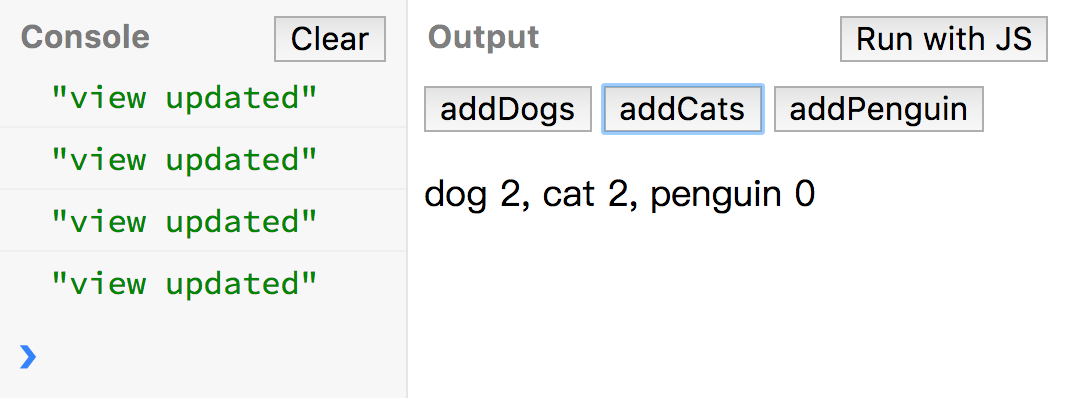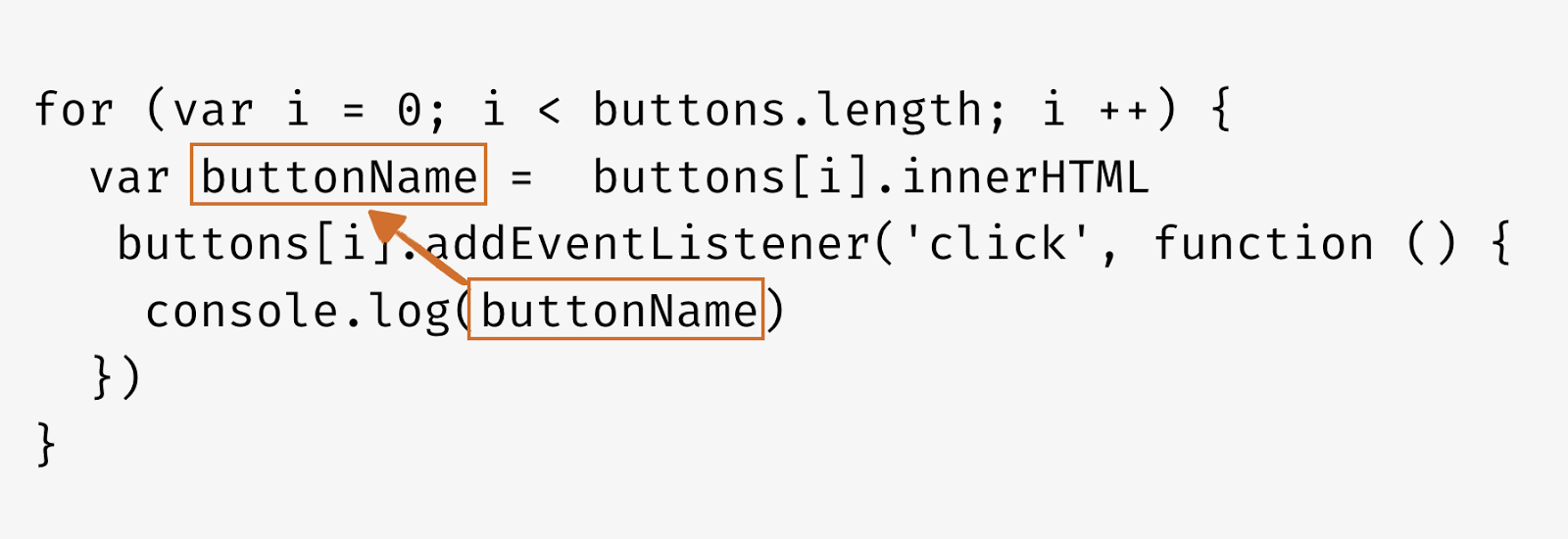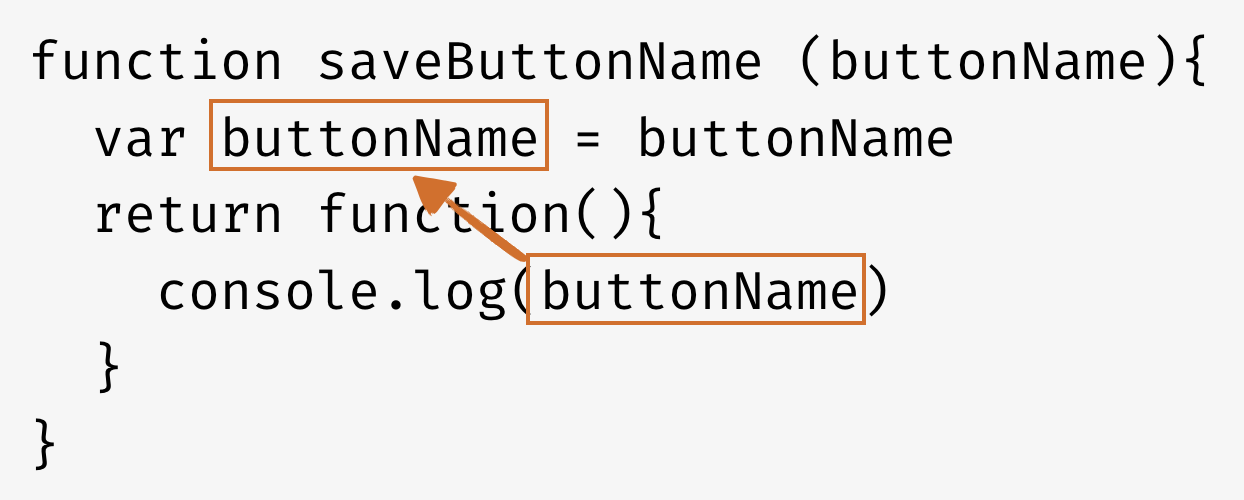# SVG 原地旋轉

SVG 物體旋轉的時候是以 <svg></svg> 的左上角當作圓心進行旋轉，因此當我們直接對 svg 內的元素進行 rotate 時，常常不是我們想要的效果。若我們想要讓物體在原地進行選轉的話，可以使用一些技巧。## 操作範例

#### STEP 0: 原本方形的位置

``````<svg>
<!--   原本方形的位置(400, 300)   -->
<rect x="400" y="300" width="40" height="30" stroke="#41b883" stroke-width="2" fill="#41CEC0"></rect>
</svg>
``````

#### STEP 1: 先讓物體進行位移（translate）把圓心移到原物體中心點``````// x' = x + width / 2
x' = 400 + 40 / 2 = 420
// y' = y + height / 2
y' = 300 + 30 / 2 = 315
``````

``````<!--  STEP1: 先移動旋轉圓心的位置    -->
<!--   x' = x + width /2  -->
<!--   y' = y + height / 2   -->
<!--   translate(400 + 40/2, 300 + 30/2)   -->
<rect x="400" y="300" width="40" height="30" stroke="#41b883" stroke-width="2" fill="#41CEC0" transform="translate(420, 315)"></rect>
``````

#### STEP 2: 選擇想要旋轉的角度

``````<!--   STEP2: 進行旋轉   -->
<!--   rotate(45)   -->
<rect x="400" y="300" width="40" height="30" stroke="#41b883" stroke-width="2" fill="#41CEC0" transform="translate(420, 315) rotate(45)"></rect>
``````

#### STEP 3: 將方形移回原本的位置

``````<!--   STEP3: 移回原本的位置   -->
<!--   translate(-420, -315)   -->
<rect x="400" y="300" width="40" height="30" stroke="#41b883" stroke-width="2" fill="#41CEC0" transform="translate(420, 315) rotate(45) translate(-420, -315)"></rect>
``````

``````<!--   直接把旋轉中心點給在 rotate 之中   -->
<!--   rotate(45, 420, 315)   -->
<rect x="400" y="300" width="40" height="30" stroke="#41b883" stroke-width="2" fill="#41CEC0" transform=" rotate(45, 420, 315)"></rect>
``````

# [掘竅] 為什麼畫面沒有隨資料更新 - Vue 響應式原理（Reactivity）## 物件部分：一開始沒有被註冊到的物件不會響應式更新

```<!-- template -->
<p>dog {{ counter.dog }}, cat {{ counter.cat }}, penguin {{ counter.penguin }}</p>
```

```new Vue({
el: '#unregister-object',
data: {
// 一開始沒有註冊 penguin，只註冊了 dog 和 cat
counter: {
dog: 0,
cat: 0
}
},
methods: {
this.counter[name] ++
}
},
created() {
// 在 created 的時候才建立 penguin 順便設值為 0
this.counter.penguin = 0
},
updated() {
// 讓我們可以知道組件有被更新
console.log('view updated')
}
})
```### 方法一：在 data 中要把所有需要響應式的資料設定進去

```data: {
counter: {
dog: 0,
cat: 0,
penguin: 0       // 一開始就把要響應式變化的資料設定進來
}
}
```

### 方法二：動態添加新的響應式屬性

```// 也可以使用 Vue.set(object, key, value)
created() {
// 在 created 的時候才建立 penguin 順便設值為 0
// 使用 \$set 動態新增響應式組件
this.\$set(this.counter, 'penguin', 0)
}
```

### 方法三：建立新的物件

```created () {
// 透過建立新的物件來新增物件的屬性
this.counter = Object.assign({}, this.counter, {
penguin: 0,
dog: 5
})
}
```

## 陣列部分：利用陣列索引直接設值時

Template 如下，主要是列出一個動物清單，當我按下 Change Animal 時，它會根據我所輸入的內容以陣列索引的方式變更陣列中的資料內容。另外我們多寫了一個 forceUpdate 的按鈕，點下去之後可以強制更新 vue 組件。
```<!-- template -->
<div id="change-by-array-index" v-cloak="v-cloak">
<h3>利用索引值變更 index 0 的值</h3>
<input type="text" v-model="animal"/>
<button v-on:click="changeAnimal">Change Animal</button>
<ul>
<li v-for="item in animals" v-bind:key="item">{{ item }}</li>
</ul>
<button v-on:click="\$forceUpdate()">forceUpdate</button>
</div>
```
JS 的部分有 changeAnimal 這個 function 會把使用者填寫的資料以陣列索引的方式代入 animals
```new Vue({
el: '#change-by-array-index',
data: {
animal: '',
animals: ['<YOURAnimal>', 'cat', 'penguin', 'bird', 'rabbit']
},
methods: {
changeAnimal () {
// 當利用陣列索引直接設置值時
this.animals = this.animal
}
}
})
```

### 方法一：使用 Vue 可觀察到的陣列方法

```methods: {
changeAnimal () {
this.animals.splice(0, 1, this.animal)
}
}
```

### 方法二：使用 vm.\$set

```methods: {
changeAnimal () {
// 利用 vm.\$set(array, index, value) 方法
this.\$set(this.animals, 0, this.animal)
}
}
```

## 瞭解 Vue Reactivity 的原理

See the Pen [Demo] Vue Reactivity in Vanilla JavaScript by PJCHEN (@PJCHENder) on CodePen.

# 深入淺出瞭解 JavaScript 閉包（closure）

## 不使用閉包（closure）的情況

``````// 狗的計數程式
var count = 0

function countDogs () {
count += 1
console.log(count + ' dog(s)')
}

countDogs()    // 1 dog(s)
countDogs()    // 2 dog(s)
countDogs()    // 3 dog(s)
``````

``````// 狗的計數函式
var count = 0

function countDogs () {
count += 1
console.log(count + ' dog(s)')
}

// 中間是其他程式碼...

// 貓的計數函式
var count = 0

function countCats () {
count += 1
console.log(count + ' cat(s)')
}

countCats()    // 1 cat(s)
countCats()    // 2 cat(s)
countCats()    // 3 cat(s)

``````

``````var count = 0

function countDogs () {
count += 1
console.log(count + ' dog(s)')
}

// 中間是其他程式碼...

var count = 0

function countCats () {
count += 1
console.log(count + ' cat(s)')
}

countCats()    // 1 cat(s)
countCats()    // 2 cat(s)
countCats()    // 3 cat(s)

countDogs()    // 4 dog(s)，我希望是 1 dog(s)
countDogs()    // 5 dog(s)，我希望是 2 dog(s)

countCats()    // 6 cat(s)，我希望是 4 cat(s)
``````

## 透過閉包讓 function 能夠有 private 變數

``````function dogHouse () {
var count = 0
function countDogs () {
count += 1
console.log(count + ' dogs')
}
return countDogs
}

const countDogs = dogHouse()
countDogs()    // "1 dogs"
countDogs()    // "2 dogs"
countDogs()    // "3 dogs"
````````````function dogHouse () {
var count = 0
function countDogs () {
count += 1
console.log(count + ' dogs')
}
return countDogs
}

function catHouse () {
var count = 0
function countCats () {
count += 1
console.log(count + ' cats')
}
return countCats
}

const countDogs = dogHouse()
const countCats = catHouse()

countDogs()    // "1 dogs"
countDogs()    // "2 dogs"
countDogs()    // "3 dogs"

countCats()    // "1 cats"
countCats()    // "2 cats"

countDogs()    // "4 dogs"
``````

## 進一步瞭解和使用閉包

``````function dogHouse () {
var count = 0
function countDogs () {
count += 1
console.log(count + ' dogs')
}
return countDogs
}

// 雖然都是使用 dogHouse ，但是各是不同的執行環境
// 因此彼此的變數不會互相干擾

var countGolden = dogHouse()
var countPug = dogHouse()
var countPuppy = dogHouse()

countGolden()     // 1 dogs
countGolden()     // 2 dogs

countPug()        // 1 dogs
countPuppy()      // 1 dogs

countGolden()     // 3 dogs
countPug()        // 2 dogs

``````

## 將參數代入閉包中

``````// 透過函式的參數將值代入閉包中
function dogHouse (name) {
var count = 0
function countDogs () {
count += 1
console.log(count + ' ' + name)
}
return countDogs
}

// 同樣是使用 dogHouse 但是使用不同的參數
var countGolden = dogHouse('Golden')
var countPug = dogHouse('Pug')
var countPuppy = dogHouse('Puppy')

// 結果更清楚了
countGolden()     // 1 Golden
countGolden()     // 2 Golden

countPug()        // 1 Pug
countPuppy()      // 1 Puppy

countGolden()     // 3 Golden
countPug()        // 2 Pug
``````

## 進一步簡化程式

### 直接 return function``````function dogHouse () {
var count = 0
// 把原本 countDogs 函式改成匿名函式直接放進來
return function () {
count += 1
console.log(count + ' dogs')
}
}

function catHouse () {
var count = 0
// 把原本 countCats 函式改成匿名函式直接放進來
return function () {
count += 1
console.log(count + ' cats')
}
}
``````

``````function createCounter (name) {
var count = 0
return function () {
count++
console.log(count + ' ' + name)
}
}

const dogCounter = createCounter('dog')
const catCounter = createCounter('cat')
const birdCounter = createCounter('bird')

dogCounter()     // 1 dog
dogCounter()     // 2 dog
catCounter()     // 1 cat
catCounter()     // 2 cat
birdCounter()    // 1 brid
dogCounter()     // 3 dog
catCounter()     // 3 cat
``````

## 閉包的實際應用

``````<button id="first">First</button>
<button id="second">Second</button>
<button id="third">Third</button>
``````

``````var buttons = document.getElementsByTagName('button')

for (var i = 0; i < buttons.length; i ++) {
// buttonName 暴露於 global environment
var buttonName =  buttons[i].innerHTML
console.log(buttonName)
})
}
````````````// 建立一個閉包把資料存在這個 function 當中
function saveButtonName (buttonName){
// buttonName 被儲存在閉包當中
var buttonName = buttonName
return function(){
console.log(buttonName)
}
}

var buttons = document.getElementsByTagName('button')
for (var i = 0; i < buttons.length; i ++) {
var buttonName =  buttons[i].innerHTML
}
``````### 使用 let

``````// 使用 ES6 寫法
for (var i = 0; i < buttons.length; i ++) {
let buttonName =  buttons[i].innerHTML
}
``````

[筆記] JavaScript ES6 中使用block-scoped 的 let 宣告變數

# 那些關於 Vue 的小細節 - Computed 中 getter 和 setter 觸發的時間點

## computed 的基本觀念

```    ```
new Vue({
computed: {
computedData: function () {
return // ...
}
}
})
```
```

```    ```
new Vue({
computed: {
computedData: {
get: function () {
return // ...
},
set: function () {
// ...
}
}
}
})
```
```

## 一般情況下 getter 觸發的時間點

```    ```

<!-- template -->
<div id="computed-basic" v-cloak="v-cloak">
<h3 class="title-border">一般情況 computed getter 被觸發的時間點</h3>
<form class="pure-form">
<input type="text" v-model="firstName"/>
<input type="text" v-model="lastName"/>
</form>
<p>{{fullName}}</p>
</div>

```
```
```    ```
// js: computed-basic
new Vue({
el: '#computed-basic',
data: {
firstName: 'PJ',
lastName: 'Chen'
},
computed: {
fullName () {
console.log('computed getter')
return this.firstName + ' ' + this.lastName
}
},
updated () {
console.log('updated')
}
})
```
```## getter 的例外情況：資料變更但 getter 不會被觸發

```    ```
<p> firstName: {{ firstName }}, lastName: {{ lastName }} </p>
```
```## 一般情況下 setter 觸發的時間點

``````
<!-- template -->
<div id="computed-setter-basic" v-cloak="v-cloak">
<h3 class="title-border">一般情況 computed setter 被觸發的時間點</h3>
<form class="pure-form">
<input type="text" v-model="fullName"/>
</form>
<p>firstName: {{ firstName }} <br/> lastName: {{ lastName }}</p>
</div>
```
```
``````

// js
// computed-setter-basic
new Vue({
el: '#computed-setter-basic',
data: {
firstName: 'PJ',
lastName: 'Chen'
},
computed: {
fullName: {
get () {
console.log('computed getter')
return this.firstName + ' ' + this.lastName
},
set (value) {
console.log('computed setter')
this.firstName = value.split(' ')
this.lastName = value.split(' ')
}
}
},
updated () {
console.log('updated')
}
})
```
```
template 中，我們可以看到，我們的 input 是直接綁 v-model="fullName" ，因此他會直接去修改 fullName 的值，而當前 fullName 是 computed 中的一個屬性，我們說過 computed 中的屬性就和 data 類似可以取值（getter）和設值（setter），這時候因為我們要對 fullName 設值，自然就會對應到 fullName 裡面的 setter（如果沒有設定 setter 是無法對 fullName 設值的）。## 觸發 setter 不必然會觸發 getter

``````
set (value) {
console.log('computed setter')
// this.firstName = value.split(' ')
// this.lastName = value.split(' ')
}
``````## 總結

1. getter 和 setter 彼次觸發的時間點是獨立的。 getter 在大部分的時候是當內部觀察的資料有改變時會被觸發；setter 則是當被觀察的物件本身有改變時會被觸發。
2. getter 在畫面中沒有使用到被觀察的物件時，不會被觸發。

5/10 更新，感謝網友@Aysh Su ( 聖涵 / 亞所 ) 修正觀念錯誤